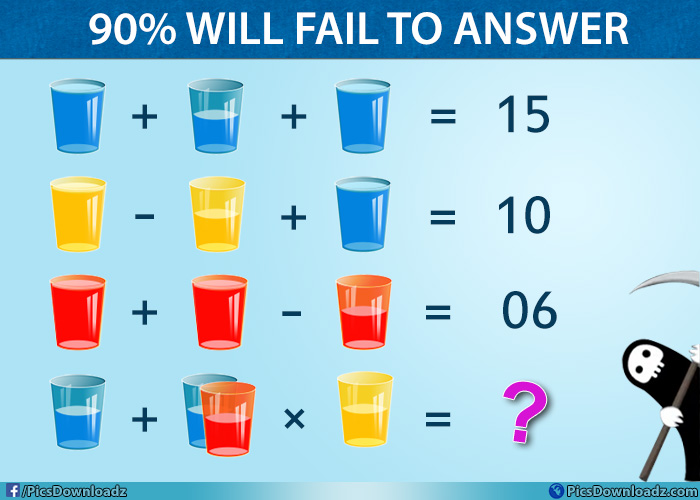This post may contain affiliate links. This means if you click on the link and purchase the item, We will receive an affiliate commission at no extra cost to you. See Our Affiliate Policy for more info.

The Red, Blue and Yellow Drinking Glass Puzzle – Genius Math Puzzle

4
5521

Solve this red, blue and yellow glass puzzle if you are a genius! Only for genius math puzzles with the answer!!

The red, blue & yellow drinking glass math puzzle. Find the values of these glasses and solve the last equation. Share your answer in comment section below. Good luck!

Drinking Glass Genius Math Puzzle Image:Got it? Comment below or keep scrolling for solution

.

A

N

S

W

E

R

.

Don’t forget to like us for more interesting updates. And share this puzzle with your friends on facebook, let see if they can crack this puzzle or not. Enjoy!

I’m sure you figured out the hack of the full glass and half glass. Now all you have to do is just find out the values of these drinking glasses.

Solution:

Consider:

Blue Glass = B Full  and B Half

Yellow Glass = Y Full and Y Half

Red Glass = R Full and R Half

Simple Method: Just consider a number for a glass and apply on equations and find out if they are equals. Suppose, if you consider a number 4 for a glass, obviously the half one will be 2 and now solve the equation, the result should be equals to the given number on the right-hand side. In this way, you can figure out the values of each color glasses.

Now put those value in the fourth equation and find out the answer. [Check the 4th equation below]

Mathematical Method:

Now let’s solve the puzzle

1st Equation:

⇒ B Full + B Half + B Full = 15

If Full Glass = B, then Half Glass = B/2

⇒ B + B/2 + B = 15

(To cancle out the half, multiply the above equation with 2 and we will get)

⇒ 2B + B + 2B = 15 × 2

⇒ 5B = 30

⇒ B = 30/5 = 6

Therefore:  B Full = 6,  so B Half = 3

2nd Equation:

⇒ Y Full – Y Half + B Full = 10

⇒ Y – Y/2 + B = 10

⇒ Y – Y/2  = 10 – B  (Put the value of B)

⇒ Y – Y/2  = 10 – 6

⇒ Y – Y/2 = 4

⇒ 2Y – Y = 4 × 2

⇒ Y = 8

⇒ So, Y Full = 8   and   Y Half = 4

3rd Equation:

⇒ R + R – (R/2) = 06

⇒ 2R + 2R – R = 06 × 2

⇒ 4R – R = 12

⇒ 3R = 12

⇒ R = 12/3 = 4

So, R Full = 4 and R Half = 2

Now we have the value of Red, Blue and Yellow Glass:

Blue: B Full = 6   and   B Half = 3

Yellow: Y Full = 8   and   Y Half = 4

Red:  R Full= 4  and R Half = 2

4th Equation:

⇒  B Half + (B Half + R Half) × Y Half = ??

⇒  3 + (3 + 2) × 4 = ??

⇒  3 + (5) × 4 = ??

⇒  3 + 20 = ??

Like our Page:

Search items:

Viral Math Puzzles, Only for geniuses puzzles, Viral Facebook Math Puzzles, Puzzles for Kids, Teach Math with Puzzles, Solve this Genius Puzzles Image, Drinking Glass Math Puzzle, Maths Puzzles with Answer, Confusing Math Puzzle Image, Fun Math Puzzle Problems, Difficult Math Puzzle Images, Fun Math Puzzle Pictures, Share Puzzle on Facebook, Whatsapp puzzle image, Genius Math Riddles

1.swarnalatha

•Kumar

2.sutarman
3.Turny